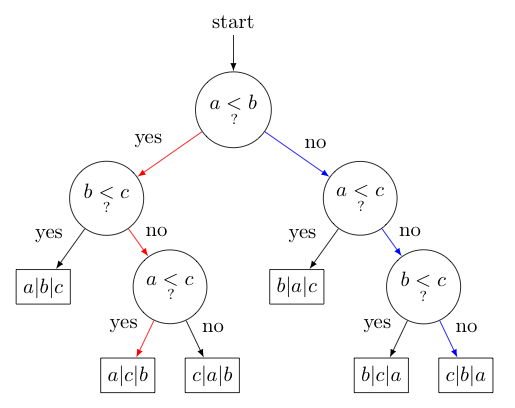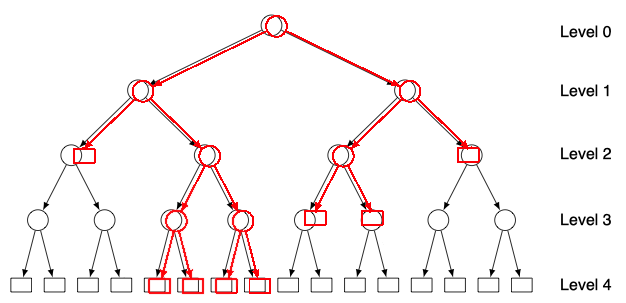## Decision Trees: Finding a Lower Bound on Comparison Cost

We have now looked at a number of different sorting algorithms. Some are clearly better than others in certain contexts. For example, selection and insertion sorts tend to require many more comparisons as $n$ increases than do quicksorts and merge sorts. One naturally wonders if there is some other sorting algorithm that does even better than quicksort or merge sort. How small can we make the comparison cost for a sorting method? Is there a limit?

As it turns out, there is indeed a lower bound on the comparison cost for sorting algorithms that rely solely on pairwise comparing elements to decide the next steps to take (which includes all of the sorting techniques we have so far described). The best we can ever do is $O(n \ln n)$. Why you ask? Consider the following:

Let us consider an insertion sort on an array of just three values: $$\begin{array}{|c|c|c|}\hline a & b & c \\\hline \hline \end{array}$$ Let us further suppose that $a \lt c \lt b$. In conducting an insertion sort we make several comparisons between pairs of elements. For this particular case, we have listed below each comparison in the order they were made, and the corresponding changes in the array that resulted:

$$\begin{array}{ccc} a \lt b? & (yes) & \begin{array}{|c|c|c|}\hline a & b & c\\\hline\hline\end{array}\\ b \lt c? & (no) & \begin{array}{|c|c|c|}\hline a & c & b\\\hline\hline\end{array}\\ a \lt c? & (yes) & \begin{array}{|c|c|c|}\hline a & c & b\\\hline\hline\end{array}\\ \end{array}$$

Of course, if the true order of $a$, $b$, $c$ had been different, different comparisons might have been made, leading to different results. For example, if $c \lt b \lt a$, then we would have seen these comparisons: $$\begin{array}{ccc} a \lt b? & (no) & \begin{array}{|c|c|c|}\hline b & a & c\\\hline\hline\end{array}\\ a \lt c? & (no) & \begin{array}{|c|c|c|}\hline b & c & a\\\hline\hline\end{array}\\ b \lt c? & (no) & \begin{array}{|c|c|c|}\hline c & b & a\\\hline\hline\end{array}\\ \end{array}$$

There are a total of $3! = 6$ possible orderings of $a$, $b$, and $c$. The flowchart below, which is called a decision tree, compactly describes the comparisons made and the final array states associated with all $6$ possibilities. Notice how the red and blue paths describe the comparisons made in the first and second example above, respectively.Take a moment and convince yourself that this particular organization of comparisons and arrays results from the use of an insertion sort on each possible ordering of $a$, $b$, and $c$. Upon doing so, you should see that we could build a decision tree corresponding to any comparison-based sorting algorithm on $n$ elements.

Note that $\fbox{$a|c|b$}$ appears on level 3 (the top-most comparison is traditionally considered level 0). Consequently, when $a \lt c \lt b$, sorting $\{a,b,c\}$ involves 3 comparisons. Similarly, $\fbox{$b|a|c$}$ appears on level 2, and when $b \lt a \lt c$, only 2 comparisons are needed to sort $a,b,c$. With this in mind, it should be clear from the picture above that the maximum number of comparisons we need to worry about when sorting 3 elements with an insertion sort corresponds to the highest level of any of the arrays shown.

Extending this idea, the more levels a decision tree has, the more comparisons we may need to make to sort any given array with the sorting algorithm used to generate the tree.

So to find a lower bound on the comparison cost of any sorting algorithm, the question becomes "What's the smallest number of levels $h$, that we could have in a decision tree connected to sorting $n$ items?"

To answer this question, note first that for $n$ items, there are $n!$ possible final array orderings. This means we will need at least this many arrays at the ends of the branches (we generally call these leaves) in the corresponding decision tree. (Indeed, we might have even more if more than one path of comparisons lead to the same array.)

Of course, the number of leaves for a tree of $h$ levels is at most $2^h$, obtained by adding all possible branches so that every leaf is on level $h$. To see this, consider the overlayed images of a red and gray tree below. Both have the same number of levels, but the gray tree has a maximum number of leaves for that many levels. Looking at this gray tree, note that there is $2^0=1$ circle on level $0$, $2^1=2$ circles on level $1$, $2^2=4$ circles on level $2$, $2^3=8$ on level $3$, and finally $2^4=16$ leaves on level $4$.As such, $$n! \le \textrm{number of leaves} \le 2^h$$ Taking $\log_2$ of both sides gives us $$\log_2 (n!) \le h$$ Consequently, no comparison-based sorting algorithm can guarantee to sort $n$ items with less than $\log_2 (n!)$ comparisons!

We have established the desired lower bound, but it will be useful to go one more step and apply Stirling's Approximation, which tells us $\log_2 n! \sim n \log_2 n$. (We won't prove Stirling's result, as the above argument gives us plenty to digest already -- but the curious reader can find a proof here, if desired.)

Knowing the number of comparisons required by any comparison-based sorting algorithm is $\Omega(n \log_2 n)$ allows us to better see how the comparison cost functions for the sorting algorithms already discussed stack up against this ideal.